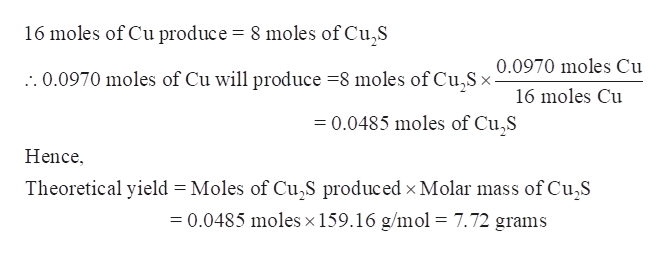# When copper is heated with an excess of sulfur, copper(I) sulfide is formed. In a given experiment, 0.0970 moles of copper was heated with excess sulfur to yield 3.46 g copper(I) sulfide. What is the percent yield?

Question
18 views

When copper is heated with an excess of sulfur, copper(I) sulfide is formed. In a given experiment, 0.0970 moles of copper was heated with excess sulfur to yield 3.46 g copper(I) sulfide. What is the percent yield?

check_circle

Step 1

When copper is heated with an excess of sulfur, copper (I) sulfide is formed. In a given experiment, 0.0970 moles of copper were heated with excess sulfur to yield 3.46 g copper (I) sulfide. The percent yield is to be determined.

Step 2

Write the chemical equation showing the reaction of copper with excess of sulfur.

Step 3

Determine the theoretical yield of copper (I) sulfide-

As per the balanced equation, 16 moles of Cu yield 8 moles of c...help_outlineImage Transcriptionclose16 moles of Cu produce = 8 moles of Cu,S 0.0970 moles Cu :.0.0970 moles of Cu will produce 8 moles of Cu,Sx- 16 moles Cu 0.0485 moles of Cu2S Hence Theoretical yield Moles of Cu,S produced x Molar mass of Cu2S 0.0485 moles x 159.16 g/mol = 7.72 grams fullscreen

### Want to see the full answer?

See Solution

#### Want to see this answer and more?

Solutions are written by subject experts who are available 24/7. Questions are typically answered within 1 hour.*

See Solution
*Response times may vary by subject and question.
Tagged in

### General Chemistry